[This article was first published on R – Daniel Oehm | Gradient Descending, and kindly contributed to R-bloggers]. (You can report issue about the content on this page here)
Want to share your content on R-bloggers? click here if you have a blog, or here if you don't.Every so often a problem arises where it’s appropriate to use gradient descent, and it’s fun (and / or easier) to apply it manually. Recently I’ve applied it optimising a basic recommender system to ‘unsuppressing’ suppressed tabular data. I thought I’d do a series of posts about how I’ve used gradient descent, but figured it was worth while starting with the basics as a primer / refresher.

# Linear regression

To understand how this works gradient descent is applied we’ll use the classic example, linear regression.

A simple linear regression model is of the form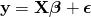where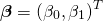The objective is to find the parameterssuch that they minimise the mean squared error.This is a good problem since we know the analytical solution and can check our results.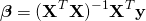In practice you would never use gradient descent to solve a regression problem, but it is useful for learning the concepts.

# Example data

Set up

library(ggplot2)
set.seed(241)

nobs <- 250
b0 <- 4
b1 <- 2

# simulate data
x <- rnorm(nobs)
y <- b0 + b1*x + rnorm(nobs, 0, 0.5)
df <- data.frame(x, y)

# plot data
g1 <- ggplot(df, aes(x = x, y = y)) +
geom_point(size = 2) +
theme_minimal()

The analytical solution is given by

# set model matrix
X <- model.matrix(y ~ x, data = df)
beta <- solve(t(X) %*% X) %*% t(X) %*% y
beta

##                 [,1]
## (Intercept) 4.009283
## x           2.016444

And just to convince ourselves this is correct

# linear model formulation
lm1 <- lm(y ~ x, data = df)
coef(lm1)

## (Intercept)           x
##    4.009283    2.016444

g1 + geom_abline(slope = coef(lm1), intercept = coef(lm1), col = "darkmagenta", size = 1)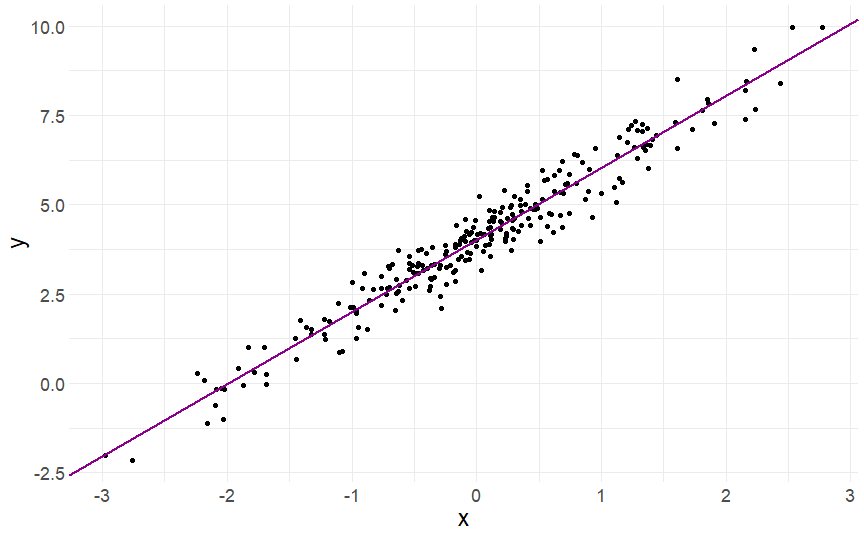The objective is to achieve the same result using gradient descent. It works by updating the parameters with each iteration in the direction of negative gradient to minimise the mean squared error i.e.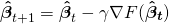where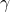is the learning rate. Hereis the MSE with respect to the regression parameters. Firstly, we find the partial derivatives of.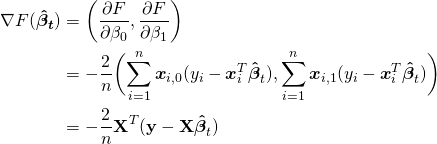The learning rate is to ensure we don’t jump too far with each iteration and rather some proportion of the gradient, otherwise we could end up overshooting the minimum and taking much longer to converge or not find the optimal solution at all.

Applying this to the problem above, we’ll initialise our values forto something sensible e.g.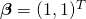. I’ll choose a learning rate of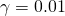. This is a slow burn, a learning rate of 0.1-0.2 is more appropriate for this problem but we’ll get to see the movement of the gradient better. It’s worth trying different values ofto see how it changes convergence. The algorithm is setup as

# gradient descent function
gradientDescent <- function(formula, data, par.init, loss.fun, lr, iters){
formula <- as.formula(formula)
X <- model.matrix(formula, data = data)
y <- data[,all.vars(formula)]
par <- loss <- matrix(NA, nrow = iters+1, ncol = 2)
par[1,] <- par.init
for(k in 1:iters){
loss[k,] <- loss.fun(X=X, y=y, par=par[k,])
par[k+1,] <- par[k,] - lr*loss[k,]
}
return(list(par = par))
}

# loss function
loss.fun <- function(X, y, par) return(-2/nrow(X)*(t(X) %*% (y - X %*% par)))

# gradient descent. not much to it really
beta <- gradientDescent(y ~ x, data = df, par.init = c(1, 1), loss.fun = loss.fun, lr = 0.01, iters = 1000)$par # plotting results z <- seq(1, 1001, 10) g1 + geom_abline(slope = beta[z,2], intercept = beta[z,1], col = "darkmagenta", alpha = 0.2, size = 1)tail(beta, 1) ## [,1] [,2] ## [1001,] 4.009283 2.016444 As expected we obtain the same result. The lines show the gradient and how the parameters converge to the optimal values. A less reasonable set of starting values still converges quickly to the optimal solution showing how well graident descent works on linear regression. beta <- gradientDescent(y ~ x, data = df, par.init = c(6, -1), loss.fun = loss.fun, lr = 0.01, iters = 1000)$par

# plotting results
z <- seq(1, 1001, 10)
beta.df <- data.frame(b0 = beta[z,1], b1 = beta[z,2])
g1 + geom_abline(data = beta.df, mapping = aes(slope = b1, intercept = b0), col = "darkmagenta", alpha = 0.2, size = 1)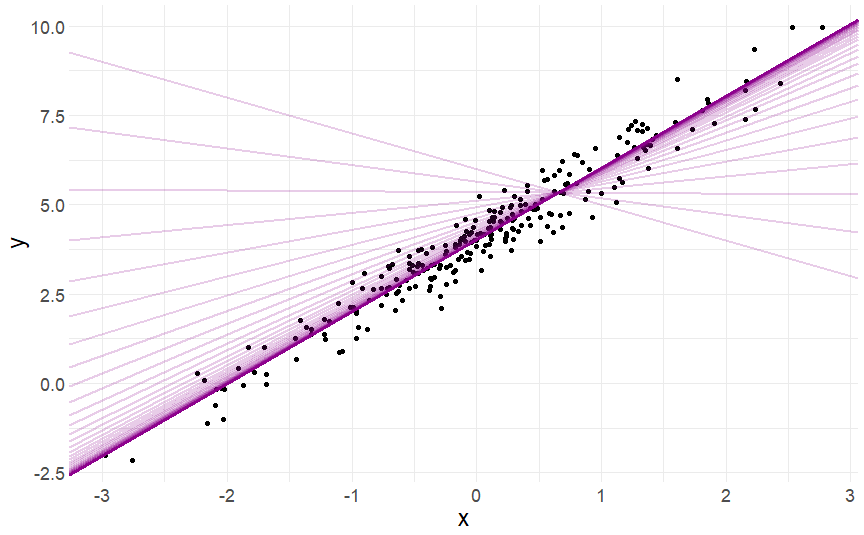tail(beta, 1)

##             [,1]     [,2]
## [1001,] 4.009283 2.016444

ggif_minimal <- df %>%
ggplot(aes(x = x, y = y)) +
geom_point(size = 2) +
theme_minimal() +
geom_abline(data = beta.df, mapping = aes(slope = b1, intercept = b0), col = "darkmagenta", size = 1) +
geom_text(
data = data.frame(z, b0 = beta[z,1], b1 = beta[z,2]),
mapping = aes(
x = -2.8, y = 9,
label = paste("b0 = ", round(b0, 2), "\nb1 = ", round(b1, 2))),
hjust = 0,
size = 6
) +
transition_reveal(z) +
ease_aes("linear") +
enter_appear() +

animate(ggif_minimal, width = 1920, height = 1080, fps = 80)

# Takeaways

They are the basics of applying gradient descent. In practice there is no need to use gradient descent to solve a regression problem, but once you know how to apply it you’ll find real-world applications elsewhere that are more complicated (and interesting). If you can define the objective function and it is differentiable, you can apply gradient descent. In later posts i’ll demonstrate how I’ve applied it to real world problems. Stay tuned!

The post Applying gradient descent – primer / refresher appeared first on Daniel Oehm | Gradient Descending.